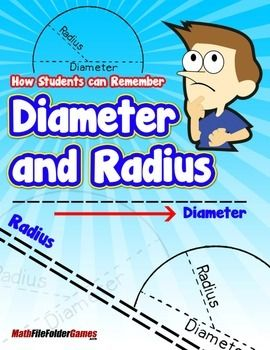# How Students Can Remember Diameter and RadiusHow Students can Remember Diameter and Radius

Students have a hard time remembering which line is the diameter in a circle, and which is the radius.

Show them this memory trick: when written alongside each other, “d” (diameter) is twice as tall as “r” (radius)!

This reminds them that the diameter of a circle is twice the length of the radius.